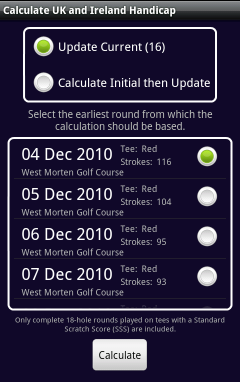# Calculating a UK/IRL handicap.

A UK/IRL handicap for a player may be calculated from within the 'Edit Players' screen.

Note: Despite using the approach published by CONGU, the handicap calculated should not be considered as official and should be used as a guide only.

At least 3 valid 18-hole rounds must have been recorded in order to calculate a UK/IRL handicap.

Click the appropriate button on the 'Edit Players' screen and a screen will displayed showing the valid rounds recorded for the player.From the rounds displayed, the first round to be included in the calculation should be selected. For example, if 10 rounds are displayed but, for some reason, it is required that the calculation should only include the data from the last 5 rounds then the radio button against the 6th round should be selected.

To calculate an initial handicap value, at least 2 valid rounds following the selected round must be available (3 in total). If more than 3 rounds are available then the subsequent rounds are used to calculate an updated value to the initial handicap.

An update to an existing handicap requires that at least 1 valid round (in total) is available.

Following the calculation of a handicap, it is possible to view the steps taken to achieve the result.To calculate an initial handicap, the first three rounds are used. For each round, the number of strokes is adjusted so that it is no more than 2 over par. The total of these values provides the Adjusted Total for the round (shown as Adjd.).

The SSS is then subtracted from the Adjusted Total to give the Gross value and finally, the initial handicap is calculated as the average of the 3 Gross values.

When updating a handicap, each round (excluding those used to generate an initial handicap) is processed in turn and may cause the handicap to go up, down or stay the same.

To calculate the impact of a single round on the current handicap value:

1. The number of strokes for each hole is adjusted so that it is no more than 2 over par. The total of these values provides the Adjusted Total (Adjd.).
2. The SSS is subtracted from the Adjusted Total to give the Gross value.
3. The player's current handicap (at this point in the calculation) is subtracted from the Gross value to give the Net value.
4. The player's current handicap also identifies the buffer zone category to be used which in turn defines if and by how much the handicap should be increased or reduced depending on the Net value for the round.
5. If the Net value is within the buffer zone (i.e. between zero and the upper limit) then the handicap remains the same, if above the upper limit then the handicap is increased by a single amount, or if the Net value is below zero then the handicap is reduced by a set amount per stroke below zero.
CategoryHandicap
Range
Buffer
Zone
Reduction
(per stroke)
Increase
10.1-5.4+1-0.10.1
25.5-12.4+2-0.20.1
312.5-20.4+3-0.30.1
420.5-28.4+4-0.40.1
5>=28.5+5-0.50